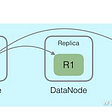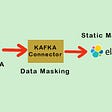# 1. Recursion

## Scenario I. a Large Variety of Unique Values Are Available (1 Million Unique Values)

`\timing  drop table test;  create unlogged table test(id int , info text, crt_time timestamp);`
`insert into test select ceil(random()*1000000), md5(random()::text), clock_timestamp() from generate_series(1,5000000);`
`create index idx_test_1 on test (id, crt_time desc);`
`explain (analyze,verbose,timing,costs,buffers) with recursive skip as (      (        select test as v from test where id in (select id from test where id is not null order by id,crt_time desc limit 1) limit 1    )      union all      (        select (        select t as v from test t where t.id>(s.v).id and t.id is not null order by id,crt_time desc limit 1      ) from skip s where (s.v).id is not null    )      -- "where (s.v).id is not null" must be added, otherwise it will end up with an endless loop.   )     select (t.v).id, (t.v).info, (t.v).crt_time from skip t where t.* is not null;                                                                                         QUERY PLAN                                                                                        --------------------------------------------------------------------------------------------------------------------------------------------------------------------------------------   CTE Scan on skip t  (cost=54.35..56.37 rows=100 width=44) (actual time=0.042..6626.084 rows=993288 loops=1)     Output: (t.v).id, (t.v).info, (t.v).crt_time     Filter: (t.* IS NOT NULL)     Rows Removed by Filter: 1     Buffers: shared hit=3976934     CTE skip       ->  Recursive Union  (cost=0.91..54.35 rows=101 width=69) (actual time=0.034..6006.615 rows=993289 loops=1)             Buffers: shared hit=3976934             ->  Limit  (cost=0.91..0.93 rows=1 width=69) (actual time=0.033..0.033 rows=1 loops=1)                   Output: test.*                   Buffers: shared hit=8                   ->  Nested Loop  (cost=0.91..10.19 rows=500 width=69) (actual time=0.032..0.032 rows=1 loops=1)                         Output: test.*                         Buffers: shared hit=8                         ->  HashAggregate  (cost=0.48..0.49 rows=1 width=4) (actual time=0.021..0.021 rows=1 loops=1)                               Output: test_1.id                               Group Key: test_1.id                               Buffers: shared hit=4                               ->  Limit  (cost=0.43..0.47 rows=1 width=12) (actual time=0.016..0.016 rows=1 loops=1)                                     Output: test_1.id, test_1.crt_time                                     Buffers: shared hit=4                                     ->  Index Only Scan using idx_test_1 on public.test test_1  (cost=0.43..173279.36 rows=5000002 width=12) (actual time=0.015..0.015 rows=1 loops=1)                                           Output: test_1.id, test_1.crt_time                                           Index Cond: (test_1.id IS NOT NULL)                                           Heap Fetches: 1                                           Buffers: shared hit=4                         ->  Index Scan using idx_test_1 on public.test  (cost=0.43..9.64 rows=6 width=73) (actual time=0.009..0.009 rows=1 loops=1)                               Output: test.*, test.id                               Index Cond: (test.id = test_1.id)                               Buffers: shared hit=4             ->  WorkTable Scan on skip s  (cost=0.00..5.14 rows=10 width=32) (actual time=0.006..0.006 rows=1 loops=993289)                   Output: (SubPlan 1)                   Filter: ((s.v).id IS NOT NULL)                   Rows Removed by Filter: 0                   Buffers: shared hit=3976926                   SubPlan 1                     ->  Limit  (cost=0.43..0.49 rows=1 width=81) (actual time=0.005..0.005 rows=1 loops=993288)                           Output: t_1.*, t_1.id, t_1.crt_time                           Buffers: shared hit=3976926                           ->  Index Scan using idx_test_1 on public.test t_1  (cost=0.43..102425.17 rows=1666667 width=81) (actual time=0.005..0.005 rows=1 loops=993288)                                 Output: t_1.*, t_1.id, t_1.crt_time                                 Index Cond: ((t_1.id > (s.v).id) AND (t_1.id IS NOT NULL))                                 Buffers: shared hit=3976926   Planning time: 0.354 ms   Execution time: 6706.105 ms  (45 rows)`

## Scenario 2: A Small Number of Unique Values (1,000 Unique Values)

`\timing  drop table test;  create unlogged table test(id int , info text, crt_time timestamp);`
`insert into test select ceil(random()*1000), md5(random()::text), clock_timestamp() from generate_series(1,5000000);`
`create index idx_test_1 on test (id, crt_time desc);`
`The query statement stays unchanged  QUERY PLAN                                                                                        --------------------------------------------------------------------------------------------------------------------------------------------------------------------------------------   CTE Scan on skip t  (cost=55.09..57.11 rows=100 width=44) (actual time=0.046..8.859 rows=1000 loops=1)     Output: (t.v).id, (t.v).info, (t.v).crt_time     Filter: (t.* IS NOT NULL)     Rows Removed by Filter: 1     Buffers: shared hit=4007     CTE skip       ->  Recursive Union  (cost=0.91..55.09 rows=101 width=69) (actual time=0.039..8.203 rows=1001 loops=1)             Buffers: shared hit=4007             ->  Limit  (cost=0.91..1.67 rows=1 width=69) (actual time=0.038..0.038 rows=1 loops=1)                   Output: test.*                   Buffers: shared hit=8                   ->  Nested Loop  (cost=0.91..6335.47 rows=8333 width=69) (actual time=0.038..0.038 rows=1 loops=1)                         Output: test.*                         Buffers: shared hit=8                         ->  HashAggregate  (cost=0.48..0.49 rows=1 width=4) (actual time=0.021..0.021 rows=1 loops=1)                               Output: test_1.id                               Group Key: test_1.id                               Buffers: shared hit=4                               ->  Limit  (cost=0.43..0.47 rows=1 width=12) (actual time=0.016..0.017 rows=1 loops=1)                                     Output: test_1.id, test_1.crt_time                                     Buffers: shared hit=4                                     ->  Index Only Scan using idx_test_1 on public.test test_1  (cost=0.43..173279.55 rows=5000002 width=12) (actual time=0.015..0.015 rows=1 loops=1)                                           Output: test_1.id, test_1.crt_time                                           Index Cond: (test_1.id IS NOT NULL)                                           Heap Fetches: 1                                           Buffers: shared hit=4                         ->  Index Scan using idx_test_1 on public.test  (cost=0.43..6284.98 rows=5000 width=73) (actual time=0.015..0.015 rows=1 loops=1)                               Output: test.*, test.id                               Index Cond: (test.id = test_1.id)                               Buffers: shared hit=4             ->  WorkTable Scan on skip s  (cost=0.00..5.14 rows=10 width=32) (actual time=0.008..0.008 rows=1 loops=1001)                   Output: (SubPlan 1)                   Filter: ((s.v).id IS NOT NULL)                   Rows Removed by Filter: 0                   Buffers: shared hit=3999                   SubPlan 1                     ->  Limit  (cost=0.43..0.49 rows=1 width=81) (actual time=0.007..0.007 rows=1 loops=1000)                           Output: t_1.*, t_1.id, t_1.crt_time                           Buffers: shared hit=3999                           ->  Index Scan using idx_test_1 on public.test t_1  (cost=0.43..102425.80 rows=1666667 width=81) (actual time=0.007..0.007 rows=1 loops=1000)                                 Output: t_1.*, t_1.id, t_1.crt_time                                 Index Cond: ((t_1.id > (s.v).id) AND (t_1.id IS NOT NULL))                                 Buffers: shared hit=3999   Planning time: 0.353 ms   Execution time: 8.980 ms  (45 rows)`

## Scenario 1: A Large Number of Unique Values (1 Million Unique Values)

`explain (analyze,verbose,timing,costs,buffers) select (select test from test where id=t.id order by crt_time desc limit 1) from generate_series(1,1000000) t(id);                                                                   QUERY PLAN                                                                    ---------------------------------------------------------------------------------------------------------------------------------------------   Function Scan on pg_catalog.generate_series t  (cost=0.00..1976.65 rows=1000 width=32) (actual time=70.682..2835.109 rows=1000000 loops=1)     Output: (SubPlan 1)     Function Call: generate_series(1, 1000000)     Buffers: shared hit=3997082     SubPlan 1       ->  Limit  (cost=0.43..1.97 rows=1 width=77) (actual time=0.002..0.002 rows=1 loops=1000000)             Output: test.*, test.crt_time             Buffers: shared hit=3997082             ->  Index Scan using idx_test_1 on public.test  (cost=0.43..9.64 rows=6 width=77) (actual time=0.002..0.002 rows=1 loops=1000000)                   Output: test.*, test.crt_time                   Index Cond: (test.id = t.id)                   Buffers: shared hit=3997082   Planning time: 0.119 ms   Execution time: 2892.712 ms  (14 rows)`

## Scenario 2: A Small Number of Unique Values (1,000 Unique Values)

`explain (analyze,verbose,timing,costs,buffers) select (select test from test where id=t.id order by crt_time desc limit 1) from generate_series(1,1000) t(id);                                                                     QUERY PLAN                                                                     ------------------------------------------------------------------------------------------------------------------------------------------------   Function Scan on pg_catalog.generate_series t  (cost=0.00..1699.41 rows=1000 width=32) (actual time=0.107..7.041 rows=1000 loops=1)     Output: (SubPlan 1)     Function Call: generate_series(1, 1000)     Buffers: shared hit=4000     SubPlan 1       ->  Limit  (cost=0.43..1.69 rows=1 width=77) (actual time=0.006..0.007 rows=1 loops=1000)             Output: test.*, test.crt_time             Buffers: shared hit=4000             ->  Index Scan using idx_test_1 on public.test  (cost=0.43..6284.98 rows=5000 width=77) (actual time=0.006..0.006 rows=1 loops=1000)                   Output: test.*, test.crt_time                   Index Cond: (test.id = t.id)                   Buffers: shared hit=4000   Planning time: 0.131 ms   Execution time: 7.126 ms  (14 rows)`

# 2. Window Query

## Scenario 1: A Large Number of Unique Values (1 Million Unique Values)

`explain (analyze,verbose,timing,costs,buffers) select id,info,crt_time from (select row_number() over (partition by id order by crt_time desc) as rn, * from test) t where rn=1;  postgres=# explain (analyze,verbose,timing,costs,buffers) select id,info,crt_time from (select row_number() over (partition by id order by crt_time desc) as rn, * from test) t where rn=1;                                                                         QUERY PLAN                                                                          ---------------------------------------------------------------------------------------------------------------------------------------------------------   Subquery Scan on t  (cost=0.43..310779.41 rows=25000 width=45) (actual time=0.027..6398.308 rows=993288 loops=1)     Output: t.id, t.info, t.crt_time     Filter: (t.rn = 1)     Rows Removed by Filter: 4006712     Buffers: shared hit=5018864     ->  WindowAgg  (cost=0.43..248279.39 rows=5000002 width=53) (actual time=0.026..5973.497 rows=5000000 loops=1)           Output: row_number() OVER (?), test.id, test.info, test.crt_time           Buffers: shared hit=5018864           ->  Index Scan using idx_test_1 on public.test  (cost=0.43..160779.35 rows=5000002 width=45) (actual time=0.019..4058.476 rows=5000000 loops=1)                 Output: test.id, test.info, test.crt_time                 Buffers: shared hit=5018864   Planning time: 0.121 ms   Execution time: 6446.901 ms  (13 rows)`

## Scenario 2: A Small Number of Unique Values (1,000 Unique Values)

`The query statement stays unchanged                                                                         QUERY PLAN                                                                          ---------------------------------------------------------------------------------------------------------------------------------------------------------   Subquery Scan on t  (cost=0.43..310779.61 rows=25000 width=45) (actual time=0.027..6176.801 rows=1000 loops=1)     Output: t.id, t.info, t.crt_time     Filter: (t.rn = 1)     Rows Removed by Filter: 4999000     Buffers: shared hit=4744850 read=18157     ->  WindowAgg  (cost=0.43..248279.58 rows=5000002 width=53) (actual time=0.026..5822.576 rows=5000000 loops=1)           Output: row_number() OVER (?), test.id, test.info, test.crt_time           Buffers: shared hit=4744850 read=18157           ->  Index Scan using idx_test_1 on public.test  (cost=0.43..160779.55 rows=5000002 width=45) (actual time=0.020..4175.082 rows=5000000 loops=1)                 Output: test.id, test.info, test.crt_time                 Buffers: shared hit=4744850 read=18157   Planning time: 0.108 ms   Execution time: 6176.924 ms  (13 rows)`

# Summary

--

--

--

## More from Alibaba Cloud

Follow me to keep abreast with the latest technology news, industry insights, and developer trends. Alibaba Cloud website:https://www.alibabacloud.com

Love podcasts or audiobooks? Learn on the go with our new app.

## Our experience as an Upwork client, the story so far## How to Backup Google Big Query## How Do SMEs Efficiently Develop Software at Home## iMessage App Extenstion## Build Mobile App Fast And Easy with Expo## Elasticsearch Distributed Consistency Principles Analysis (3) — Data## Alibaba Cloud

Follow me to keep abreast with the latest technology news, industry insights, and developer trends. Alibaba Cloud website:https://www.alibabacloud.com

## Deploying Airflow in Local Kubernetes Cluster: Part II## How to Connect Elastic Sink Connector with Kafka## Historize elastic APM server data